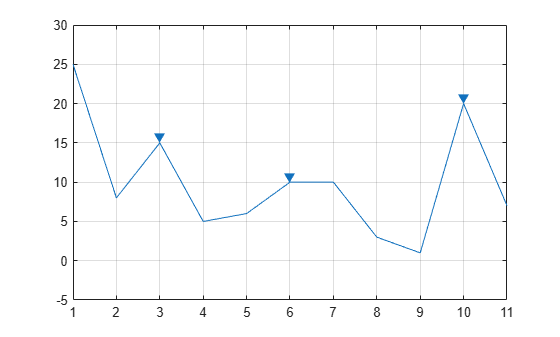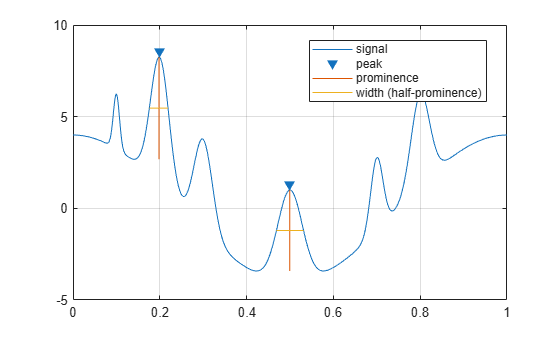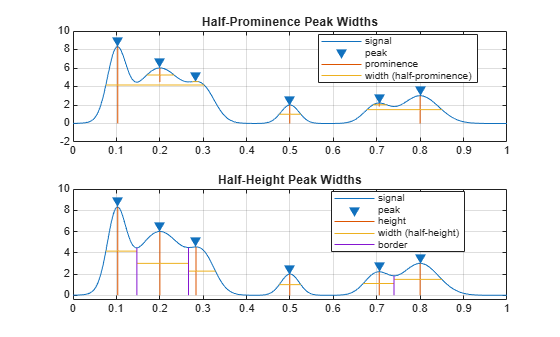# findpeaks

Find local maxima

## Syntax

``pks = findpeaks(data)``
``[pks,locs] = findpeaks(data)``
``````[pks,locs,w,p] = findpeaks(data)``````
``[___] = findpeaks(data,x)``
``[___] = findpeaks(data,Fs)``
``[___] = findpeaks(___,Name,Value)``
``findpeaks(___)``

## Description

example

````pks = findpeaks(data)` returns a vector with the local maxima (peaks) of the input signal vector, `data`. A local peak is a data sample that is either larger than its two neighboring samples or is equal to `Inf`. Non-`Inf` signal endpoints are excluded. If a peak is flat, the function returns only the point with the lowest index.```

example

````[pks,locs] = findpeaks(data)` additionally returns the indices at which the peaks occur.```

example

``````[pks,locs,w,p] = findpeaks(data)``` additionally returns the widths of the peaks as the vector `w` and the prominences of the peaks as the vector `p`.```

example

````[___] = findpeaks(data,x)` specifies `x` as the location vector and returns any of the output arguments from previous syntaxes. `locs` and `w` are expressed in terms of `x`.```

example

````[___] = findpeaks(data,Fs)` specifies the sample rate, `Fs`, of the data. The first sample of `data` is assumed to have been taken at time zero. `locs` and `w` are converted to time units.```

example

````[___] = findpeaks(___,Name,Value)` specifies options using name-value pair arguments in addition to any of the input arguments in previous syntaxes.```

example

````findpeaks(___)` without output arguments plots the signal and overlays the peak values.```

## Examples

collapse all

Define a vector with three peaks and plot it.

```data = [25 8 15 5 6 10 10 3 1 20 7]; plot(data)```Find the local maxima. The peaks are output in order of occurrence. The first sample is not included despite being the maximum. For the flat peak, the function returns only the point with lowest index.

`pks = findpeaks(data)`
```pks = 1×3 15 10 20 ```

Use `findpeaks` without output arguments to display the peaks.

`findpeaks(data)`Create a signal that consists of a sum of bell curves. Specify the location, height, and width of each curve.

```x = linspace(0,1,1000); Pos = [1 2 3 5 7 8]/10; Hgt = [3 4 4 2 2 3]; Wdt = [2 6 3 3 4 6]/100; for n = 1:length(Pos) Gauss(n,:) = Hgt(n)*exp(-((x - Pos(n))/Wdt(n)).^2); end PeakSig = sum(Gauss);```

Plot the individual curves and their sum.

`plot(x,Gauss,'--',x,PeakSig)`Use `findpeaks` with default settings to find the peaks of the signal and their locations.

`[pks,locs] = findpeaks(PeakSig,x);`

Plot the peaks using `findpeaks` and label them.

```findpeaks(PeakSig,x) text(locs+.02,pks,num2str((1:numel(pks))'))```Sort the peaks from tallest to shortest.

```[psor,lsor] = findpeaks(PeakSig,x,'SortStr','descend'); findpeaks(PeakSig,x) text(lsor+.02,psor,num2str((1:numel(psor))'))```Create a signal that consists of a sum of bell curves riding on a full period of a cosine. Specify the location, height, and width of each curve.

```x = linspace(0,1,1000); base = 4*cos(2*pi*x); Pos = [1 2 3 5 7 8]/10; Hgt = [3 7 5 5 4 5]; Wdt = [1 3 3 4 2 3]/100; for n = 1:length(Pos) Gauss(n,:) = Hgt(n)*exp(-((x - Pos(n))/Wdt(n)).^2); end PeakSig = sum(Gauss)+base;```

Plot the individual curves and their sum.

`plot(x,Gauss,'--',x,PeakSig,x,base)`Use `findpeaks` to locate and plot the peaks that have a prominence of at least 4.

`findpeaks(PeakSig,x,'MinPeakProminence',4,'Annotate','extents')`The highest and lowest peaks are the only ones that satisfy the condition.

Display the prominences and the widths at half prominence of all the peaks.

```[pks,locs,widths,proms] = findpeaks(PeakSig,x); widths```
```widths = 1×6 0.0154 0.0431 0.0377 0.0625 0.0274 0.0409 ```
`proms`
```proms = 1×6 2.6816 5.5773 3.1448 4.4171 2.9191 3.6363 ```

Sunspots are a cyclic phenomenon. Their number is known to peak roughly every 11 years.

Load the file `sunspot.dat`, which contains the average number of sunspots observed every year from 1700 to 1987. Find and plot the maxima.

```load sunspot.dat year = sunspot(:,1); avSpots = sunspot(:,2); findpeaks(avSpots,year)```Improve your estimate of the cycle duration by ignoring peaks that are very close to each other. Find and plot the peaks again, but now restrict the acceptable peak-to-peak separations to values greater than six years.

`findpeaks(avSpots,year,'MinPeakDistance',6)`Use the peak locations returned by `findpeaks` to compute the mean interval between maxima.

```[pks,locs] = findpeaks(avSpots,year,'MinPeakDistance',6); meanCycle = mean(diff(locs))```
```meanCycle = 10.9600 ```

Create a `datetime` array using the year data. Assume the sunspots were counted every year on March 20th, close to the vernal equinox. Find the peak sunspot years. Use the `years` function to specify the minimum peak separation as a `duration`.

```ty = datetime(year,3,20); [pk,lk] = findpeaks(avSpots,ty,'MinPeakDistance',years(6)); plot(ty,avSpots,lk,pk,'o')```Compute the mean sunspot cycle using `datetime` functionality.

`dttmCycle = years(mean(diff(lk)))`
```dttmCycle = 10.9600 ```

Create a timetable with the data. Specify the time variable in years. Plot the data. Show the last five entries of the timetable.

```TT = timetable(years(year),avSpots); plot(TT.Time,TT.Variables)````entries = TT(end-4:end,:)`
```entries=5×1 timetable Time avSpots ________ _______ 1983 yrs 66.6 1984 yrs 45.9 1985 yrs 17.9 1986 yrs 13.4 1987 yrs 29.3 ```

Load an audio signal sampled at 7418 Hz. Select 200 samples.

```load mtlb select = mtlb(1001:1200);```

Find the peaks that are separated by at least 5 ms.

To apply this constraint, `findpeaks` chooses the tallest peak in the signal and eliminates all peaks within 5 ms of it. The function then repeats the procedure for the tallest remaining peak and iterates until it runs out of peaks to consider.

`findpeaks(select,Fs,'MinPeakDistance',0.005)`Find the peaks that have an amplitude of at least 1 V.

`findpeaks(select,Fs,'MinPeakHeight',1)`Find the peaks that are at least 1 V higher than their neighboring samples.

`findpeaks(select,Fs,'Threshold',1)`Find the peaks that drop at least 1 V on either side before the signal attains a higher value.

`findpeaks(select,Fs,'MinPeakProminence',1)`Sensors can return clipped readings if the data are larger than a given saturation point. You can choose to disregard these peaks as meaningless or incorporate them to your analysis.

Generate a signal that consists of a product of trigonometric functions of frequencies 5 Hz and 3 Hz embedded in white Gaussian noise of variance 0.1². The signal is sampled for one second at a rate of 100 Hz. Reset the random number generator for reproducible results.

```rng default fs = 1e2; t = 0:1/fs:1-1/fs; s = sin(2*pi*5*t).*sin(2*pi*3*t)+randn(size(t))/10;```

Simulate a saturated measurement by truncating every reading that is greater than a specified bound of 0.32. Plot the saturated signal.

```bnd = 0.32; s(s>bnd) = bnd; plot(t,s) xlabel('Time (s)')```Locate the peaks of the signal. `findpeaks` reports only the rising edge of each flat peak.

```[pk,lc] = findpeaks(s,t); hold on plot(lc,pk,'x')```Use the `'Threshold'` name-value pair to exclude the flat peaks. Require a minimum amplitude difference of $1{0}^{-4}$ between a peak and its neighbors.

```[pkt,lct] = findpeaks(s,t,'Threshold',1e-4); plot(lct,pkt,'o','MarkerSize',12)```Create a signal that consists of a sum of bell curves. Specify the location, height, and width of each curve.

```x = linspace(0,1,1000); Pos = [1 2 3 5 7 8]/10; Hgt = [4 4 2 2 2 3]; Wdt = [3 8 4 3 4 6]/100; for n = 1:length(Pos) Gauss(n,:) = Hgt(n)*exp(-((x - Pos(n))/Wdt(n)).^2); end PeakSig = sum(Gauss);```

Plot the individual curves and their sum.

```plot(x,Gauss,'--',x,PeakSig) grid```Measure the widths of the peaks using the half prominence as reference.

`findpeaks(PeakSig,x,'Annotate','extents')`Measure the widths again, this time using the half height as reference.

```findpeaks(PeakSig,x,'Annotate','extents','WidthReference','halfheight') title('Signal Peak Widths')```## Input Arguments

collapse all

Input data, specified as a vector. `data` must be real and must have at least three elements.

Data Types: `double` | `single`

Locations, specified as a vector or a `datetime` array. `x` must increase monotonically and have the same length as `data`. If `x` is omitted, then the indices of `data` are used as locations.

Data Types: `double` | `single` | `datetime`

Sample rate, specified as a positive scalar. The sample rate is the number of samples per unit time. If the unit of time is seconds, the sample rate has units of hertz.

Data Types: `double` | `single`

### Name-Value Pair Arguments

Specify optional comma-separated pairs of `Name,Value` arguments. `Name` is the argument name and `Value` is the corresponding value. `Name` must appear inside quotes. You can specify several name and value pair arguments in any order as `Name1,Value1,...,NameN,ValueN`.

Example: `'SortStr','descend','NPeaks',3` finds the three tallest peaks of the signal.

Maximum number of peaks to return, specified as the comma-separated pair consisting of `'NPeaks'` and a positive integer scalar. `findpeaks` operates from the first element of the input data and terminates when the number of peaks reaches the value of `'NPeaks'`.

Data Types: `double` | `single`

Peak sorting, specified as the comma-separated pair consisting of `'SortStr'` and one of these values:

• `'none'` returns the peaks in the order in which they occur in the input data.

• `'ascend'` returns the peaks in ascending or increasing order, from the smallest to the largest value.

• `'descend'` returns the peaks in descending order, from the largest to the smallest value.

Minimum peak height, specified as the comma-separated pair consisting of `'MinPeakHeight'` and a real scalar. Use this argument to have `findpeaks` return only those peaks higher than `'MinPeakHeight'`. Specifying a minimum peak height can reduce processing time.

Data Types: `double` | `single`

Minimum peak prominence, specified as the comma-separated pair consisting of `'MinPeakProminence'` and a real scalar. Use this argument to have `findpeaks` return only those peaks that have a relative importance of at least `'MinPeakProminence'`. For more information, see Prominence.

Data Types: `double` | `single`

Minimum height difference between a peak and its neighbors, specified as the comma-separated pair consisting of `'Threshold'` and a nonnegative real scalar. Use this argument to have `findpeaks` return only those peaks that exceed their immediate neighboring values by at least the value of `'Threshold'`.

Data Types: `double` | `single`

Minimum peak separation, specified as the comma-separated pair consisting of `'MinPeakDistance'` and a positive real scalar. When you specify a value for `'MinPeakDistance'`, the algorithm chooses the tallest peak in the signal and ignores all peaks within `'MinPeakDistance'` of it. The function then repeats the procedure for the tallest remaining peak and iterates until it runs out of peaks to consider.

• If you specify a location vector, `x`, then `'MinPeakDistance'` must be expressed in terms of `x`. If `x` is a `datetime` array, then specify `'MinPeakDistance'` as a `duration` scalar or as a numeric scalar expressed in days.

• If you specify a sample rate, `Fs`, then `'MinPeakDistance'` must be expressed in units of time.

• If you specify neither `x` nor `Fs`, then `'MinPeakDistance'` must be expressed in units of samples.

Use this argument to have `findpeaks` ignore small peaks that occur in the neighborhood of a larger peak.

Data Types: `double` | `single` | `duration`

Reference height for width measurements, specified as the comma-separated pair consisting of `'WidthReference'` and either `'halfprom'` or `'halfheight'`. `findpeaks` estimates the width of a peak as the distance between the points where the descending signal intercepts a horizontal reference line. The height of the line is selected using the criterion specified in `'WidthReference'`:

• `'halfprom'` positions the reference line beneath the peak at a vertical distance equal to half the peak prominence. See Prominence for more information.

• `'halfheight'` positions the reference line at one-half the peak height. The line is truncated if any of its intercept points lie beyond the borders of the peaks selected by setting `'MinPeakHeight'`, `'MinPeakProminence'`, and `'Threshold'`. The border between peaks is defined by the horizontal position of the lowest valley between them. Peaks with height less than zero are discarded.

The locations of the intercept points are computed by linear interpolation.

Minimum peak width, specified as the comma-separated pair consisting of `'MinPeakWidth'` and a positive real scalar. Use this argument to select only those peaks that have widths of at least `'MinPeakWidth'`.

• If you specify a location vector, `x`, then `'MinPeakWidth'` must be expressed in terms of `x`. If `x` is a `datetime` array, then specify `'MinPeakWidth'` as a `duration` scalar or as a numeric scalar expressed in days.

• If you specify a sample rate, `Fs`, then `'MinPeakWidth'` must be expressed in units of time.

• If you specify neither `x` nor `Fs`, then `'MinPeakWidth'` must be expressed in units of samples.

Data Types: `double` | `single` | `duration`

Maximum peak width, specified as the comma-separated pair consisting of `'MaxPeakWidth'` and a positive real scalar. Use this argument to select only those peaks that have widths of at most `'MaxPeakWidth'`.

• If you specify a location vector, `x`, then `'MaxPeakWidth'` must be expressed in terms of `x`. If `x` is a `datetime` array, then specify `'MaxPeakWidth'` as a `duration` scalar or as a numeric scalar expressed in days.

• If you specify a sample rate, `Fs`, then `'MaxPeakWidth'` must be expressed in units of time.

• If you specify neither `x` nor `Fs`, then `'MaxPeakWidth'` must be expressed in units of samples.

Data Types: `double` | `single` | `duration`

Plot style, specified as the comma-separated pair consisting of `'Annotate'` and one of these values:

• `'peaks'` plots the signal and annotates the location and value of every peak.

• `'extents'` plots the signal and annotates the location, value, width, and prominence of every peak.

This argument is ignored if you call `findpeaks` with output arguments.

## Output Arguments

collapse all

Local maxima, returned as a vector of signal values. If there are no local maxima, then `pks` is empty.

Peak locations, returned as a vector.

• If you specify a location vector, `x`, then `locs` contains the values of `x` at the peak indices.

• If you specify a sample rate, `Fs`, then `locs` is a numeric vector of time instants with a time difference of 1/`Fs` between consecutive samples.

• If you specify neither `x` nor `Fs`, then `locs` is a vector of integer indices.

Peak widths, returned as a vector of real numbers. The width of each peak is computed as the distance between the points to the left and right of the peak where the signal intercepts a reference line whose height is specified by `WidthReference`. The points themselves are found by linear interpolation.

• If you specify a location vector, `x`, then the widths are expressed in terms of `x`.

• If you specify a sample rate, `Fs`, then the widths are expressed in units of time.

• If you specify neither `x` nor `Fs`, then the widths are expressed in units of samples.

Peak prominences, returned as a vector of real numbers. The prominence of a peak is the minimum vertical distance that the signal must descend on either side of the peak before either climbing back to a level higher than the peak or reaching an endpoint. See Prominence for more information.

collapse all

### Prominence

The prominence of a peak measures how much the peak stands out due to its intrinsic height and its location relative to other peaks. A low isolated peak can be more prominent than one that is higher but is an otherwise unremarkable member of a tall range.

To measure the prominence of a peak:

1. Place a marker on the peak.

2. Extend a horizontal line from the peak to the left and right until the line does one of the following:

• Crosses the signal because there is a higher peak

• Reaches the left or right end of the signal

3. Find the minimum of the signal in each of the two intervals defined in Step 2. This point is either a valley or one of the signal endpoints.

4. The higher of the two interval minima specifies the reference level. The height of the peak above this level is its prominence.

`findpeaks` makes no assumption about the behavior of the signal beyond its endpoints, whatever their height. This is reflected in Steps 2 and 4 and often affects the value of the reference level. Consider for example the peaks of this signal:Peak NumberLeft Interval Lies Between Peak andRight Interval Lies Between Peak andLowest Point on the Left IntervalLowest Point on the Right IntervalReference Level (Highest Minimum)
1Left endCrossing due to peak 2Left endpointaa
2Left endRight endLeft endpointhLeft endpoint
3Crossing due to peak 2Crossing due to peak 4bcc
4Crossing due to peak 2Crossing due to peak 6bdb
5Crossing due to peak 4Crossing due to peak 6dee
6Crossing due to peak 2Right enddhd
7Crossing due to peak 6Crossing due to peak 8fgg
8Crossing due to peak 6Right endfhf
9Crossing due to peak 8Crossing due to right endpointhii